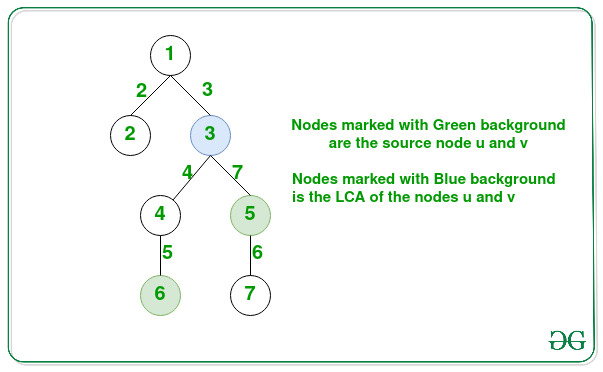Related Articles

# Maximize sum of paths from LCA of nodes u and v to one of those nodes

• Last Updated : 15 Sep, 2021

Given a tree consisting of N nodes an array edges[] of size N – 1 such that for each {X, Y, W} in edges[] there exists an edge between node X and node Y with a weight of W and two nodes u and v, the task is to find the maximum sum of weights of edges in the path from Lowest Common Ancestor(LCA) of nodes (u, v) to node u and node v

Examples:

Attention reader! Don’t stop learning now. Get hold of all the important DSA concepts with the DSA Self Paced Course at a student-friendly price and become industry ready.  To complete your preparation from learning a language to DS Algo and many more,  please refer Complete Interview Preparation Course.

In case you wish to attend live classes with experts, please refer DSA Live Classes for Working Professionals and Competitive Programming Live for Students.

Input: N = 7, edges[][] = {{1, 2, 2}, {1, 3, 3}, {3, 4, 4}, {4, 6, 5}, {3, 5, 7}, {5, 7, 6}}, u = 6, v = 5
Output: 9
Explanation:The path sum from node 3 to node 5 is 7.
The path sum from node 3 to node 6 is 4 + 5 = 9.
Therefore, the maximum among the two paths is 9.

Input: N = 4, edges[][] = {{1, 2, 3}, {2, 3, 4}, {3, 4, 5}, u = 1, v = 4
Output: 12

Approach: The given problem can be solved by using the concept of Binary Lifting to find the LCA. Follow the steps below to solve the problem:

Below is the implementation of the above approach:

## C++

 `// C++ program for the above approach``#include ``#define ll long long int``using` `namespace` `std;``const` `ll N = 100001;``const` `ll M = log2(N) + 1;`` ` `// Keeps the track of 2^i ancestors``ll anc[N][M];`` ` `// Keeps the track of sum of path from``// 2^i ancestor to current node``ll val[N][M];`` ` `// Stores the depth for each node``ll depth[N];`` ` `// Function to build tree to find the``// LCA of the two nodes``void` `build(vector > tree[],``           ``ll x, ll p, ll w, ll d = 0)``{``    ``// Base Case``    ``anc[x] = p;``    ``val[x] = w;``    ``depth[x] = d;`` ` `    ``// Traverse the given edges[]``    ``for` `(``int` `i = 1; i < M; i++) {``        ``anc[x][i] = anc[anc[x][i - 1]][i - 1];``        ``val[x][i]``            ``= val[anc[x][i - 1]][i - 1]``              ``+ val[x][i - 1];``    ``}`` ` `    ``// Traverse the edges of node x``    ``for` `(``auto` `i : tree[x]) {``        ``if` `(i.first != p) {`` ` `            ``// Recursive Call for building``            ``// the child node``            ``build(tree, i.first, x,``                  ``i.second, d + 1);``        ``}``    ``}``}`` ` `// Function to find LCA and calculate``// the maximum distance``ll findMaxPath(ll x, ll y)``{``    ``if` `(x == y)``        ``return` `1;`` ` `    ``// Stores the path sum from LCA``    ``// to node x and y``    ``ll l = 0, r = 0;`` ` `    ``// If not on same depth, then``    ``// make the the same depth``    ``if` `(depth[x] != depth[y]) {`` ` `        ``// Find the difference``        ``ll dif = ``abs``(depth[x] - depth[y]);``        ``if` `(depth[x] > depth[y])``            ``swap(x, y);`` ` `        ``for` `(``int` `i = 0; i < M; i++) {`` ` `            ``if` `((1ll << i) & (dif)) {`` ` `                ``// Lifting y to reach the``                ``// depth of node x``                ``r += val[y][i];`` ` `                ``// Value of weights path``                ``// traversed to r``                ``y = anc[y][i];``            ``}``        ``}``    ``}`` ` `    ``// If x == y the LCA is reached,``    ``if` `(x == y)``        ``return` `r + 1;`` ` `    ``// And the maximum distance``    ``for` `(``int` `i = M - 1; i >= 0; i--) {``        ``if` `(anc[x][i] != anc[y][i]) {`` ` `            ``// Lifting both node x and y``            ``// to reach LCA``            ``l += val[x][i];``            ``r += val[y][i];``            ``x = anc[x][i];``            ``y = anc[y][i];``        ``}``    ``}``    ``l += val[x];``    ``r += val[y];`` ` `    ``// Return the maximum path sum``    ``return` `max(l, r);``}`` ` `// Driver Code``int` `main()``{``    ``// Given Tree``    ``ll N = 7;``    ``vector > tree[N + 1];`` ` `    ``tree.push_back({ 2, 2 });``    ``tree.push_back({ 1, 2 });``    ``tree.push_back({ 3, 3 });``    ``tree.push_back({ 1, 3 });``    ``tree.push_back({ 4, 4 });``    ``tree.push_back({ 3, 4 });``    ``tree.push_back({ 6, 5 });``    ``tree.push_back({ 4, 5 });``    ``tree.push_back({ 5, 7 });``    ``tree.push_back({ 3, 7 });``    ``tree.push_back({ 7, 6 });``    ``tree.push_back({ 5, 6 });`` ` `    ``// Building ancestor and val[] array``    ``build(tree, 1, 0, 0);``    ``ll u, v;``    ``u = 6, v = 5;`` ` `    ``// Function Call``    ``cout << findMaxPath(u, v);`` ` `    ``return` `0;``}`
Output:
```9
```

Time Complexity: O(N*log(N))
Auxiliary Space: O(N*log(N))

My Personal Notes arrow_drop_up